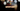# Leetcode | Solution of Cells with Odd Values in a Matrix in JavaScript

April 4th, 2020
|

In this post, we will solve Cells with Odd Values in a Matrix from leetcode and compute it's time and space complexities. Let's begin.

# Problem Statement

The question can be found at leetcode Cells with Odd Values in a Matrix problem.

The problem states that we are given size `n*m` of a matrix and some indices. The initial values at all the indices in the matrix are `0`.

Every index is in the format of `[ri,ci]` where `ri` represents all the elements of row `r` and `ci` represents all the elements of column `c`. For every index in indices, all the number of a particular row or column is increased by `1`. So, if an index is `[1,1]`, we not only increase the value at position `[1,1]` but all the elements in row `1` and all the elements in column `1`.

We need to determine the number of cells which will hold odd values in the matrix after all the indices is processed.

# Solution

One very simple solution would be to create a matrix of size `n*m` and fill it with zero. On every index loop over the entire row and column and keep on incrementing the values. Once we are done with all the values, we can loop over the matrix and find the count of odd values. Can we do better?

Yes, we can!!!. Let take a closer look and try to find a way where we don't have to create a matrix and loop over all the rows and columns multiple times.

We can have two arrays called `row` and `col` of size `n` and `m` respectively and fill it with zeroes. Now, we'll loop over the indices and for every row and column, we will increase the count at that index in array `row` and `col`. When we have looped over all the indices, we will create a nested loop and run it `n*m` times. For every value, we will find their values in array `row` and `col` and add it. If the sum is odd, we increase the count of odd values.

Why would it work? Let take an example where all the values of row `0` are increased by `1`. Instead of representing it in a matrix, we represent it in an array. Now if row `1` was increased `3` times, the position `3` in array row would be `3`. If col `4` was increased `2` times, the value in `col` array for position `4` would be `2`. So the value at position `[1,4]` would be the summation of values in array `row` and `col`. It is so because that position falls on row `1` and column `4` which has been increased those many times.

I hope I was able to clarify it. Please check the video below for more clarification.

We have discussed the approach, I urge you to go ahead on leetcode and give it another try.If you are here, it means something went wrong in implementation or you are just too lazy. In any case, let's see a simple implementation of the above logic.
``````var oddCells = function (n, m, indices) {
const row = new Array(n).fill(0)
const col = new Array(m).fill(0)

for (let i = 0; i < indices.length; i++) {
row[indices[i]]++;
col[indices[i]]++;
}

let count = 0;

for (let i = 0; i < n; i++) {
for (let j = 0; j < m; j++) {
if ((row[i] + col[j]) % 2 !== 0) {
count++;
}
}
}

return count;
};``````

The code here is the counterpart of the solution discussed above. We have created two arrays row and col of size `n` and `m` respectively.

Next, we increment the values of all the indices. At last, we find the count of odd values and return it.

Here are the stats on submission

``````Status: Accepted
Runtime: 68ms
Memory: 34.5MB``````

## Time and space complexity

### Time complexity

We are looping over all the matrix elements, so time complexity would be O(n*m).

### Space complexity

We are using extra space to store two new arrays. So space complexity would be, O(n+m). We are not exactly storing `n*m` values, we are storing only `n + m` values in two arrays.

# Summary

So, we solved the Cells with Odd Values in a Matrix problem and calculated the time and space complexities.

I hope you enjoyed solving this question. This is it for this one, complete source code for this post can be found on my Github Repo. Will see you in the next one.